Home » EOMONTH Function DAX

# EOMONTH Function DAX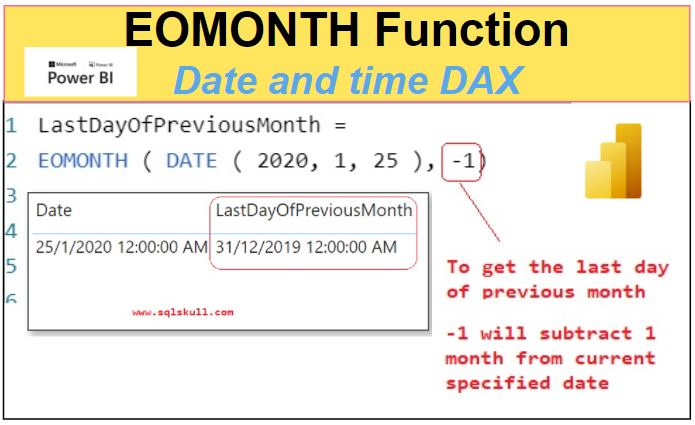EOMONTH function is a Power BI Date and time function in DAX which returns the date in datetime format of the last day of the month, before or after a specified number of months.

It returns a date in datetime format.

```SYNTAX
EOMONTH( start_date, months)```

start_date

is the start date in datetime format, EOMONTH function will return an error if start_date is not in valid date format.

months

is a number which represent the number of months before or after the start_date, If specified number is not an integer, the number is rounded up or down to the nearest integer.

#### Considerations when using EOMONTH function

• If start_date plus months evaluates an invalid date, in this case EOMONTH returns an error. start_date before March 1st of 1900 and after December 31st of 9999 are invalid.
• EOMONTH function returns an error if the text representation of the start_date is not converted to a datetime value correctly.
• If start_date is a numeric value that is not in a datetime format, EOMONTH will convert the number to a date. It is recommended to convert the number to valid date format before using to avoid invalid result.
• If start_date is a text representation of the date, the EOMONTH function uses the locale and date time settings, of the client computer, to understand the text value in order to perform the conversion. If current date time settings represent a date in the format of Month/Day/Year, then the specified string “12/6/2020” is evaluated as a datetime value equivalent to December 6th of 2020. However, if the current date time settings represent a date in the format of Day/Month/Year, the same string would be evaluated as a datetime value equivalent to June 12th of 2020.

Lets look at an example of using EOMONTH function.

Following DAX measure uses EOMONTH function which returns a last day of month for specified date Jan 25th of 2020.

You can see here we have specified a number 0 to second argument months so the EOMONTH function returns a last day of current specified date only.

```LastDayOfMonth =

EOMONTH ( DATE ( 2020, 1, 25 ), 0 )```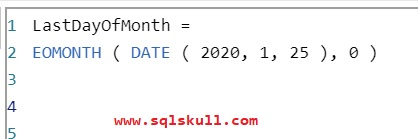Lets commit the DAX, and see the output as shown below.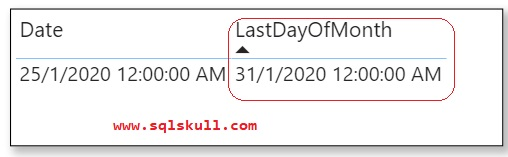You can see, it returns the last day of month that is Jan 31st 2020.

[Also Read.. date and time function DATE]

#### Get a last day of next month using EOMONTH function

Following DAX measure uses EOMONTH function which returns a last day of next for specified date Jan 25th of 2020.

You can see here we have specified a positive number 1 to second argument months so the EOMONTH function adds 1 number to the month of the current specified date and returns a last day of next month.

```LastDayOfNextMonth =

EOMONTH ( DATE ( 2020, 1, 25 ), 1)```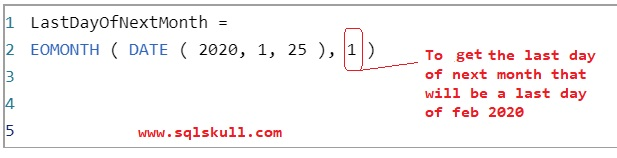Lets commit the Dax, and see the output as shown below.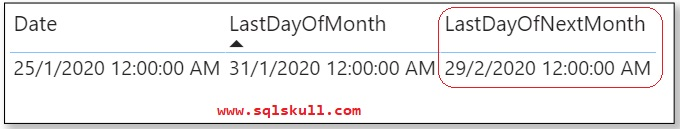You can see for a specified date Jan 25th of 2020, it returns the last day of next month that is Feb 29th of 2020.

Similarly, you can specify any positive number to see the last day of next month.

If you specify a number 2 to second argument months then it adds a 2 number to the month of current specified date and returns a last day of evaluated month that will be a march 31st 2020.

```LastDayOfNextMonth_Mar =

EOMONTH ( DATE ( 2020, 1, 25 ), 2)```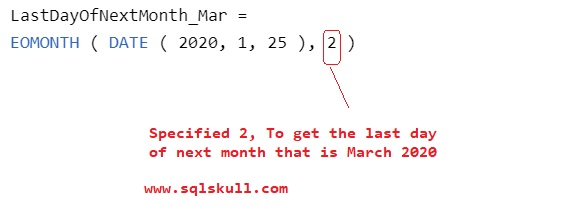You can see the output of measure as shown below.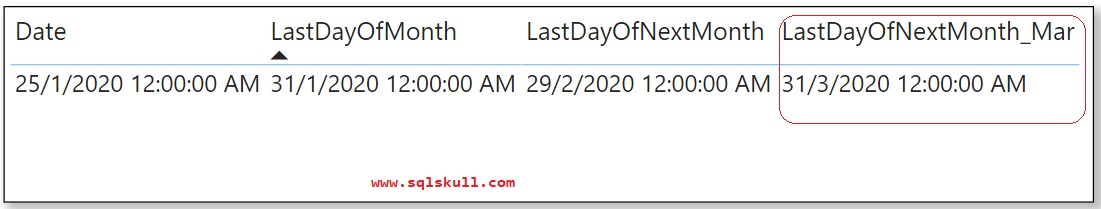#### Get a last day of previous month using EOMONTH function

Following Dax measure uses EOMONTH function, which returns a last day of previous month for specified date Jan 25th of 2020.

You can see here we have specified a negative number -1 to second argument months , which substracts 1 month from current specified date, and returns a last day of previous month.

```LastDayOfPreviousMonth =

EOMONTH ( DATE ( 2020, 1, 25 ), -1)```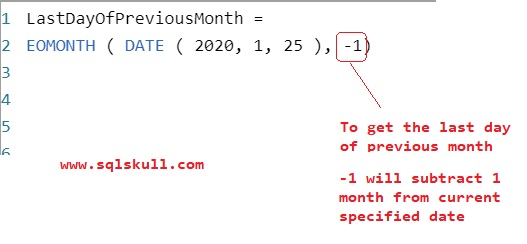Lets see the output of DAX measure.

You can see for a specified date Jan 25th of 2020, it returns the last day of previous month that is Dec 31st 2019.Similarly, you can specify any negative number such as -3,-4, .. to see the last day previous month that you desired.

Lets see a scenario when a non integer value is specified to second argument months in EOMONTH function.

If you specify non integer number lets say a positive number 2.5 to a second argument, a EOMONTH function round ups the number and make it 3.

You can see we have specified a positive number 2.5 to second argument, that will be rounded to 3 by EOMONTH function, so it adds 3 number to the month of current specified date.

```LastDayOfMonth_NonIntegerNumber =

EOMONTH ( DATE ( 2020, 1, 25 ), 2.5 )```Lets see the output of DAX measure.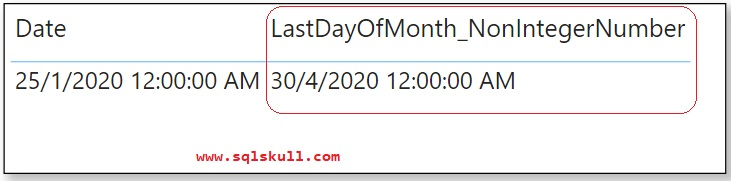You can see it returns Apr 30th of 2020, as the number 2.5 is rounded to 3,  so 3 number is added to the month of specified date.

#### Using dates before Mar 1st of 1900 and after Dec 31st of 9999 with EOMONTH Function

If start_date plus months evaluates an invalid date, in this case EOMONTH returns an error.

start_date before March 1st of 1900 and after December 31st of 9999 are invalid.

Lets see the last day of next month for date Dec 31st of 9999.

```LastDayOfMonth =

EOMONTH ( DATE ( 9999, 12, 31 ), 1 )```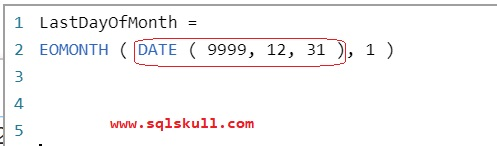Lets see the output of above DAX measure, and you can see it returns following error.